## RD Sharma Class 9 Solutions Chapter 21 Surface Areas and Volume of a Sphere VSAQS

These Solutions are part of RD Sharma Class 9 Solutions. Here we have given RD Sharma Class 9 Solutions Chapter 21 Surface Areas and Volume of a Sphere VSAQS

Other Exercises

Question 1.
Find the surface area of a sphere of radius 14 cm.
Solution:
Radius of a sphere (r) = 14 cm
∴ Surface area = 4πr2 = 4 x $$\frac { 22 }{ 7 }$$ x 14 x 14 cm2
= 2464 cm3

Question 2.
Find the total surface afea of a hemisphere of radius 10 cm.
Solution:
Radius of hemisphere (r) = 10 cm
∴ Total surface area = 3πr2Question 3.
Find the radius of a sphere whose surface area is 154 cm2.
Solution:
Surface area of a sphere = 154 cm2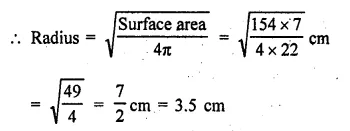Question 4.
The hollow sphere, in which the circus motor cyclist performs his stunts, has a diameter of 7 m. Find the area available to the motor cyclist for riding.
Solution:
Diameter of hollow sphere = 7 m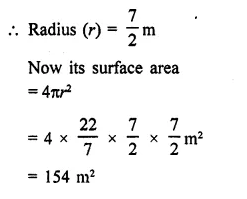Question 5.
Find the volume of a sphere whose surface area is 154 cm2.
Solution:
Surface area of a sphere = 154 cm2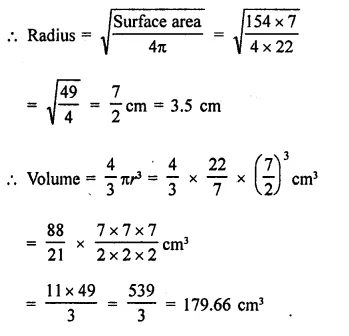Question 6.
How many spherical bullets can be made out of a solid cube of lead whose edge measures 44 cm, each bullet being 4 cm in diameter?
Solution:
Edge of a solid cube = 44 cm
∴ Volume = a2 = (44)2 cm2
= 44 × 44 × 44 cm3
Diameter of a spherical bullet = 4 cmQuestion 7.
If a sphere of radius 2r has the same volume as that of a cone with circular base of radius r, then find the height of the cone.
Solution:
Radius of a sphere (R) = 2r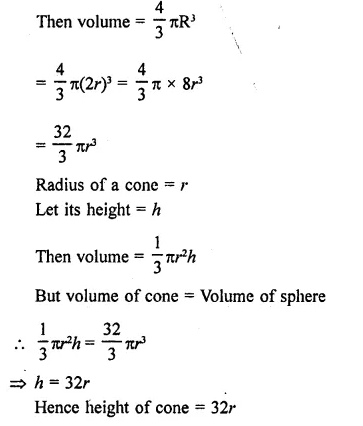Question 8.
If a hollow sphere of intefnal and external diameters 4 cm and 8 cm respectively melted into a cone of base diameter 8 cm, then find the height of the cone.
Solution:
Internal diameter of a hollow sphere = 4cm
∴ Internal radius = $$\frac { 4 }{ 2 }$$ = 2 cm
Similarly the outer radius (R) = $$\frac { 8 }{ 2 }$$ = 4 cm
∴ Volume of melted used in hollow sphereQuestion 9.
The surface area of a sphere of radius 5 cm is five times the area of the curved surface of a cone of radius 4 cm. Find the height of the cone.
Solution:
Radius of a sphere (r) = 5 cm
∴ Surface area = 4πr2
= 4π x 5 x 5 = 100π cm2
Radius of cone (r1) = 4 cm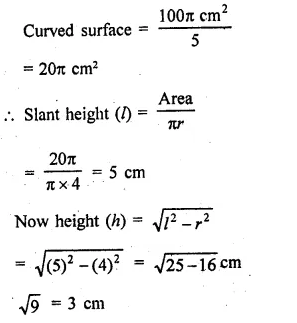Question 10.
If a sphere is inscribed in a cube, find the ratio of the volume of cube to the volume of the sphere.
Solution:
Let edge of a cube = a
Then its volume = a3
∵ A sphere is inscribed in the cube
∴ Diameter of sphere = a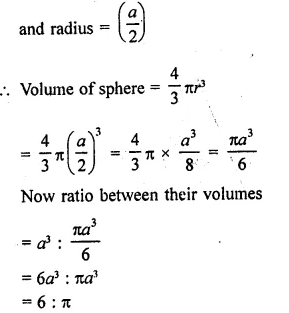Hope given RD Sharma Class 9 Solutions Chapter 21 Surface Areas and Volume of a Sphere VSAQS are helpful to complete your math homework.

If you have any doubts, please comment below. Learn Insta try to provide online math tutoring for you.